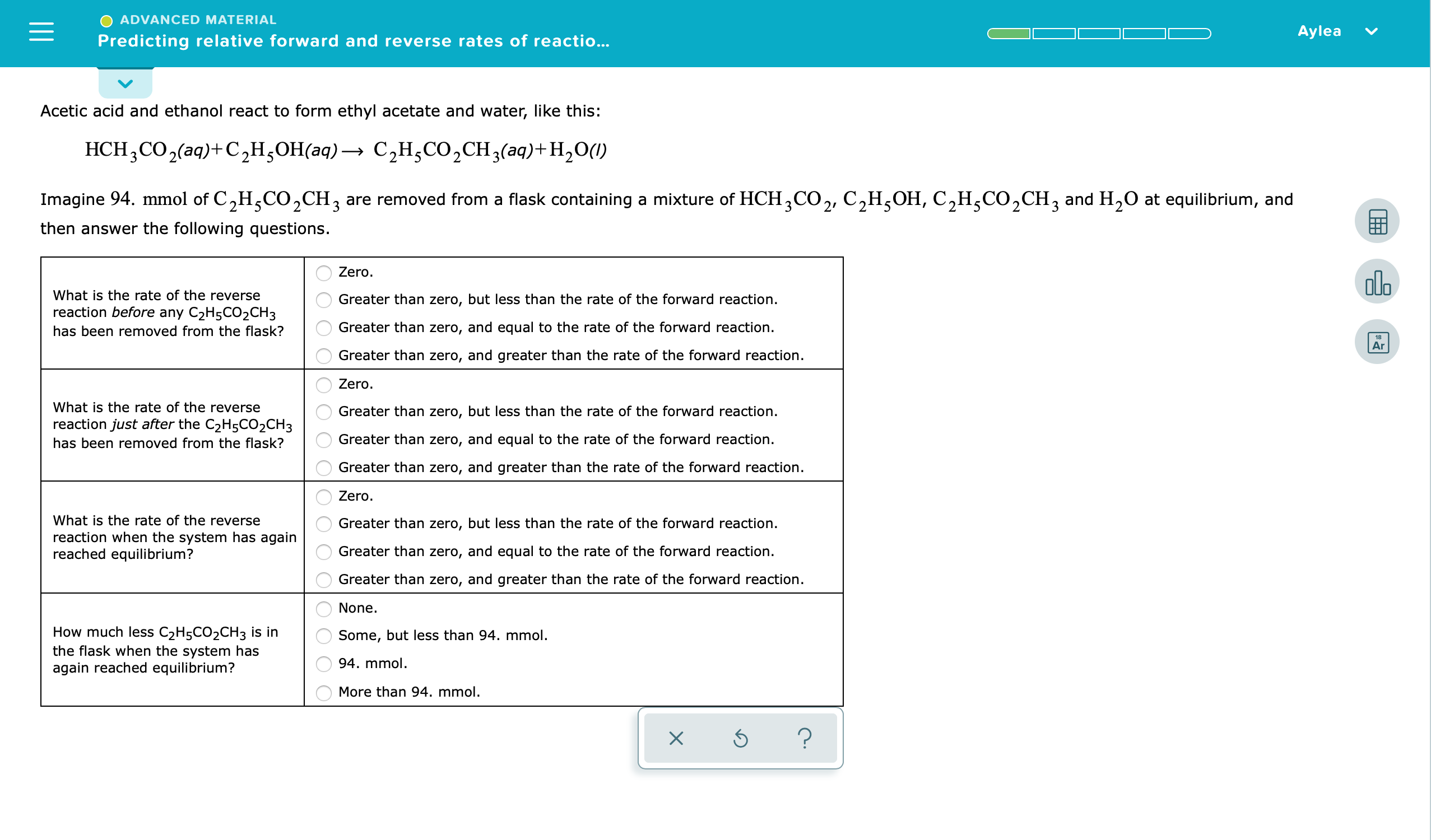# ADVANCED MATERIALAyleaPredicting relative forward and reverse rates of reactio...Acetic acid and ethanol react to form ethyl acetate and water, like this:HCH2CO2(aq)+ C2H,OH(aq) C2H,CO2CH3(aq)+H20)Imagine 94. mmol of C2H5CO2CH3 are removed from a flask containing a mixture of HCH3CO2, C2H5OH, C2H5CO2CH3 and H20 at equilibrium, andthen answer the following questions.ZeroaloWhat is the rate of the reverseGreater than zero, but less than the rate of the forward reactionreaction before any C2H5CO2CH3Greater than zero, and equal to the rate of the forward reaction.has been removed from the flask?18ArGreater than zero, and greater than the rate of the forward reactionZero.What is the rate of the reverseGreater than zero, but less than the rate of the forward reactionreaction just after the C2H5CO2CH3Greater than zero, and equal to the rate of the forward reactionhas been removed from the flask?Greater than zero, and greater than the rate of the forward reaction.ZeroWhat is the rate of the reverseGreater than zero, but less than the rate of the forward reaction.reaction when the system has againreached equilibrium?Greater than zero, and equal to the rate of the forward reaction.Greater than zero, and greater than the rate of the forward reaction.None.How much less C2H5CO2CH3 is inthe flask when the system hasagain reached equilibrium?Some, but less than 94. mmol.94. mmolMore than 94. mmol.?XOO OO O OOO

Question
143 viewshelp_outlineImage TranscriptioncloseADVANCED MATERIAL Aylea Predicting relative forward and reverse rates of reactio... Acetic acid and ethanol react to form ethyl acetate and water, like this: HCH2CO2(aq)+ C2H,OH(aq) C2H,CO2CH3(aq)+H20) Imagine 94. mmol of C2H5CO2CH3 are removed from a flask containing a mixture of HCH3CO2, C2H5OH, C2H5CO2CH3 and H20 at equilibrium, and then answer the following questions. Zero alo What is the rate of the reverse Greater than zero, but less than the rate of the forward reaction reaction before any C2H5CO2CH3 Greater than zero, and equal to the rate of the forward reaction. has been removed from the flask? 18 Ar Greater than zero, and greater than the rate of the forward reaction Zero. What is the rate of the reverse Greater than zero, but less than the rate of the forward reaction reaction just after the C2H5CO2CH3 Greater than zero, and equal to the rate of the forward reaction has been removed from the flask? Greater than zero, and greater than the rate of the forward reaction. Zero What is the rate of the reverse Greater than zero, but less than the rate of the forward reaction. reaction when the system has again reached equilibrium? Greater than zero, and equal to the rate of the forward reaction. Greater than zero, and greater than the rate of the forward reaction. None. How much less C2H5CO2CH3 is in the flask when the system has again reached equilibrium? Some, but less than 94. mmol. 94. mmol More than 94. mmol. ? X OO O O O OOO fullscreen
check_circle

Step 1

Acetic acid and ethanol react to form ethyl acetate and water.

If 94.0 mmol of C2H5CO2CH3 are removed from a flask containing a mixture of HCH3CO2, C2H5OH, C2H5CO2CH3 and H2O at equilibrium, then following questions are to be answered.

1. What is the rate of the reverse reaction before any C2H5CO2CH3 has been removed from the flask?
2. What is the rate of the reverse reaction just after the C2H5CO2CH3 has been removed from the flask?
3. What is the rate of the reverse reaction when the system has again achieved equilibrium?
4. How much less C2H5CO2CH3 is in the flask when the system has again reached equilibrium?
Step 2

The given questions are based on the Le-Chatelier’s Principle which states that-

If an established equilibrium is disturbed by changing the conditions, then the position of equilibrium changes to counteract (oppose) that change.

For instance, if the concentration of the reactant is increased, then the position of equilibrium shifts in order to decrease the concentration of reactant. That means more of reactant is to be converted into product by moving the position of equilibrium in the forward direction.

Similarly, if the concentration of the reactant is decreased, then the position of equilibrium shifts in order to increase the concentration of reactant. That means more of product is to be converted into reactants by moving the position of equilibrium in the reverse direction.

Step 3

Part 1- What is the rate of the reverse reaction before any C2H5CO2CH3 has been removed from the flask.

• Before the removal of C2H5CO2CH3 the system is in equilibrium which means that the rate of forward reaction is equal to the rate of reverse...

### Want to see the full answer?

See Solution

#### Want to see this answer and more?

Solutions are written by subject experts who are available 24/7. Questions are typically answered within 1 hour.*

See Solution
*Response times may vary by subject and question.
Tagged in

### General Chemistry